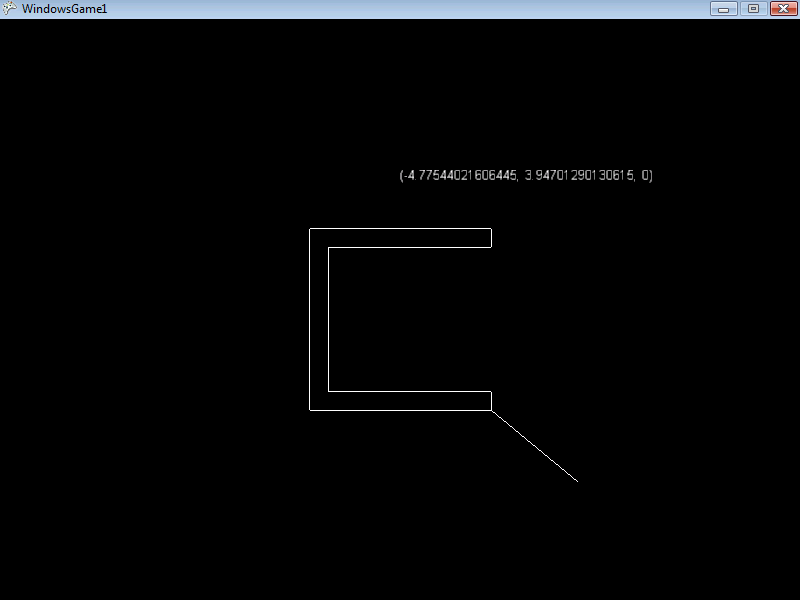# VectorFrom

## Introduction

The VectorFrom returns a vector from the argument position to the calling instance. This method returns the shortest vector which can be traveled along to reach the surface of the calling instance.

This method does not return the distance from the calling point to the center of the shape.

## Code Example

The following code creates a concave Polygon and a Line. The Line draws the shortest line from the tip of the cursor to the Polygon.

Add the following using statements:

```using FlatRedBall.Input;
using FlatRedBall.Math.Geometry;
using FlatRedBall.Graphics;
```

Add the following at class scope:

```Line connectingLine;
Polygon polygon;
Text text;
```

Add the following in Initialize after initializing FlatRedball:

```polygon = ShapeManager.AddPolygon();
Point[] points = new Point[]
{
new Point(-5, 5),
new Point(5, 5),
new Point(5, 4),
new Point(-4, 4),
new Point(-4, -4),
new Point(5, -4),
new Point(5, -5),
new Point(-5, -5),
new Point(-5, 5)
};
polygon.Points = points;
IsMouseVisible = true;
connectingLine.RelativePoint1 = new Point3D();
text.Y = 8;```

Add the following to Update:

```connectingLine.X = InputManager.Mouse.WorldXAt(0);
connectingLine.Y = InputManager.Mouse.WorldYAt(0);
Point3D vectorFrom = polygon.VectorFrom(
connectingLine.X,
connectingLine.Y);
text.DisplayText = vectorFrom.ToString();
connectingLine.RelativePoint2 = vectorFrom;```